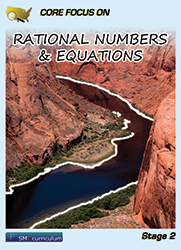#### Rational Numbers & Equations

Students apply and extend previous understandings of operations with fractions to add, subtract, multiply, and divide rational numbers.  They use properties of operations to generate equivalent expressions. Students learn to solve real-life and mathematical problems using numerical and algebraic expressions and equations.

### Textbook & Stage I Series Pricing

1 FREE Teacher Edition(TE) and Teacher Resource Binder(TR) per 40 student texts ordered!